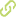# Probability distributions for Markov chain based quantum walks•
• Overview
•
• Identity
•
•
• View All
•

### Abstract

• © 2017 IOP Publishing Ltd Printed in the UK. We analyze the probability distributions of the quantum walks induced from Markov chains by Szegedy (2004). The first part of this paper is devoted to the quantum walks induced from finite state Markov chains. It is shown that the probability distribution on the states of the underlying Markov chain is always convergent in the Cesaro sense. In particular, we deduce that the limiting distribution is uniform if the transition matrix is symmetric. In the case of a non-symmetric Markov chain, we exemplify that the limiting distribution of the quantum walk is not necessarily identical with the stationary distribution of the underlying irreducible Markov chain. The Szegedy scheme can be extended to infinite state Markov chains (random walks). In the second part, we formulate the quantum walk induced from a lazy random walk on the line. We then obtain the weak limit of the quantum walk. It is noted that the current quantum walk appears to spread faster than its counterpart-quantum walk on the line driven by the Grover coin discussed in literature. The paper closes with an outlook on possible future directions.

### Publication date

• January 19, 2018